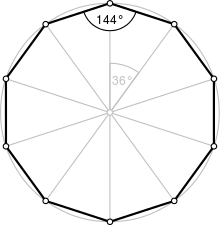# Circumscribed 22713

Calculate the circumference and the area of a regular ten-angle polygon if the radius of the circumscribed circle r = 20 cm.

S =  1175.5705 cm2
o =  123.6068 cm

### Step-by-step explanation:Did you find an error or inaccuracy? Feel free to write us. Thank you!

Tips for related online calculators
The Pythagorean theorem is the base for the right triangle calculator.